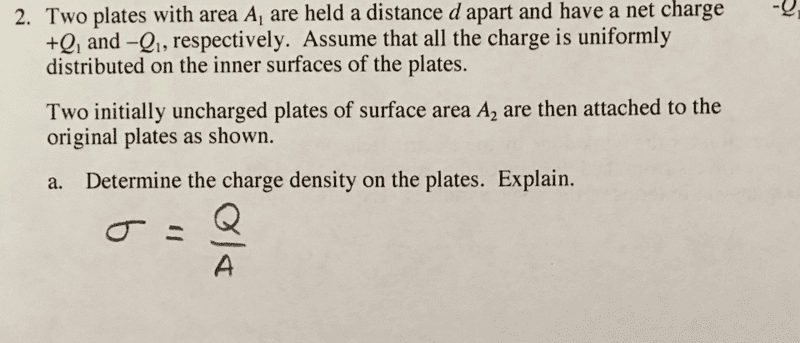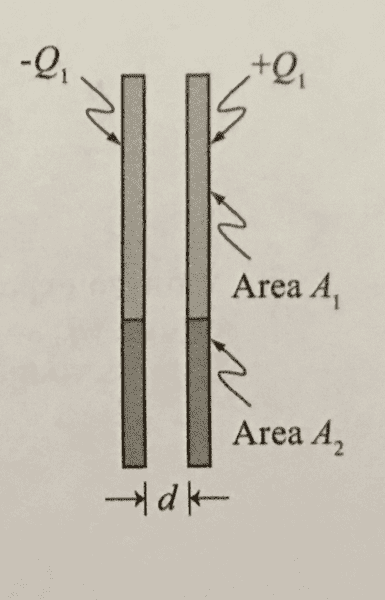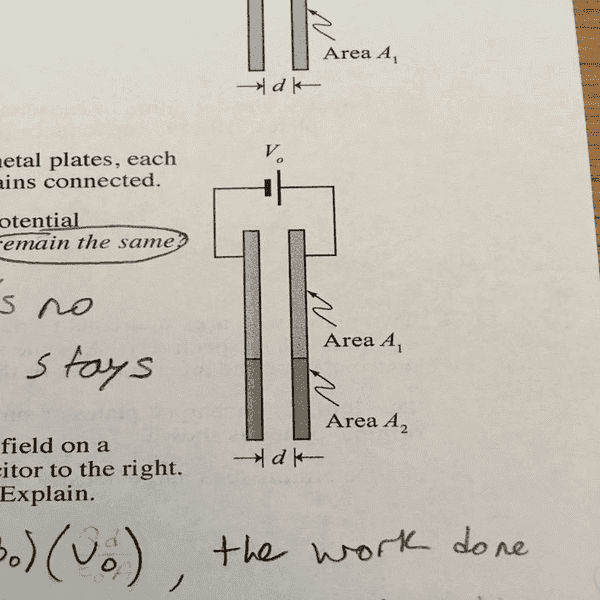# Capacitance questions

## Homework StatementWrite an expression for the total charge on one of the plates of the capacitor. Is this total charge greater than, less than, or equal to the total charge on one of the original plates?
http://imgur.com/enJ1wMy (It won't let me paste the image so this is the best I could do)

Determine the charge density on the plates. Explain.σ = Q/A
Q = C/V

## The Attempt at a Solution

For the first problem I came up with Q = C/V which is (εA/d)(Qd/εA) = Q which must be wrong so I must be mixing up equations here.

For the second question I simply said σ = Q/A since that is what we got in recitation, however, I really don't know if that is correct and why that is correct.

Thanks in advance!!

## Answers and Replies

kuruman
Science Advisor
Homework Helper
Gold Member
Can you provide the full statement of the problem? What happened to the plates that changed them from being "original"? Was just the extra area added?

On edit: OK, I got the statement now. How does the original charge on the original plates compare with the final charge on the final plates?

Can you provide the full statement of the problem? What happened to the plates that changed them from being "original"? Was just the extra area added?

On edit: OK, I got the statement now. How does the original charge on the original plates compare with the final charge on the final plates?
I want to say the original charge is less than the final plates charge since there is more area on the final plates charge compared to the initial plates. Isnt charge just Q = C/V

kuruman
Science Advisor
Homework Helper
Gold Member
I want to say the original charge is less than the final plates charge since there is more area on the final plates charge compared to the initial plates. Isnt charge just Q = C/V
Suppose what you want to say is correct. If there is more charge on the final plates than before, where did the extra charge come from?

Suppose what you want to say is correct. If there is more charge on the final plates than before, where did the extra charge come from?
Wait, that wouldn't make sense since the battery is providing a constant voltage V0 so in reality the total charge is going to be the same for both cases before and after since the battery is left unchanged correct? Cause in reality I don't know where that extra charge could come from without adding it myself which is not the case in this scenario.

kuruman
Science Advisor
Homework Helper
Gold Member
The picture of the statement of the problem that you posted says nothing about a battery being connected. Therefore, we must assume that there is no battery. So what do you think?

The picture of the statement of the problem that you posted says nothing about a battery being connected. Therefore, we must assume that there is no battery. So what do you think?
Sorry, which question are we dealing with here. The site didn't allow me to post the picture for the total charge question so I had to upload to imgur and post the link here http://imgur.com/enJ1wMy. There is supposed to be a battery for the first question and no battery for the charge density question. Sorry for any confusion I tried to post this picture, but the site constantly deleted it.For question no 2.

If battery is not connected

We can proceed like this
charge density on A1 = charge density on A2.
So let q charge displaces from A1 to A2
Charge on A1 = Q1-q
Charge on A2 = q

If battery is connected

Take ratios of two charges(initial and final) in accordance with their capacitance(initial and final).

Simply calculate total charge density using the final charge.

Let me know if u have any further doubts

Last edited:
If a battery is connected while attaching two uncharged plates of area A2. The Charge on the plates will simply increase as capacitance is increased (due to increase in area) if and only if we consider potential from battery remains constant.

For question no 2.

If battery is not connected

We can proceed like this
charge density on A1 = charge density on A2.
So let q charge displaces from A1 to A2
Charge on A1 = Q1-q
Charge on A2 = q

If battery is connected

Take ratios of two charges(initial and final) in accordance with their capacitance(initial and final).

Simply calculate total charge density using the final charge.

Let me know if u have any further doubts
So You simply take the charge density and add them together for the total charge density? Am I understanding this correctly? So instead of σ = Q/A it would be Q/(A1+A2)?

gneill
Mentor
Sorry, which question are we dealing with here. The site didn't allow me to post the picture for the total charge question so I had to upload to imgur...
Did you try the UPLOAD button in the edit pane to upload your image?Did you try the UPLOAD button in the edit pane to upload your image?

View attachment 113539
Yes, it didn't allow it for some reason. I consistently got a message saying "Security error. Please re-load the page" along those lines.

gneill
Mentor
Yes, it didn't allow it for some reason. I consistently got a message saying "Security error. Please re-load the page" along those lines.
From what site were you trying to upload? A security error usually means the file is not public accessible.

From what site were you trying to upload? A security error usually means the file is not public accessible.
No site, just my computer. I uploaded the images to imgur and from there I download them on to my computer so that I can upload the image rather than post a url. I could do the one image for question 2 but the first image would constantly have me get a Security error.

haruspex
Science Advisor
Homework Helper
Gold Member
2020 Award
take the charge density and add them together for the total charge density?
I don't think that is what you meant. What two charge densities would you be adding together?
Q/(A1+A2)?
Yes, the charge is simply spread out over a larger area.

gneill
Mentor
No site, just my computer. I uploaded the images to imgur and from there I download them on to my computer so that I can upload the image rather than post a url. I could do the one image for question 2 but the first image would constantly have me get a Security error.
Were the images all located in the same directory on your computer under the same user?

So You simply take the charge density and add them together for the total charge density? Am I understanding this correctly? So instead of σ = Q/A it would be Q/(A1+A2)?
Only if we suppose the battery is not connected.

But in case of being battery connected then

Q2/Q1 = (A1+A2)/A1
So Q2/(A1+A2) = Q1/A1
i.e. final charge density = initial charge density.

{ Q1,Q2 are supposed initial and final charge on plates respectively }